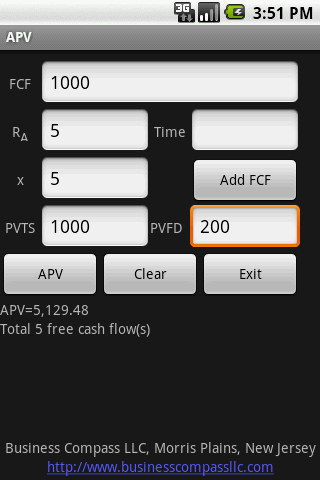# Valuation1. Adjusted Present Value (APV) from FCF, PVFD, PVTS, tax rate,Ra
2. Un-lever equity Beta from equity beta, debt beta, tax rate, debt/equity (leverage) and re-lever asset beta to debt/equity (leverage) to derive equity beta
3. Weighted average Cost of Capital (WACC)
4. Weighted average Cost of Capital Valuation

### App Specification

• App Name: Valuation
• Rating: